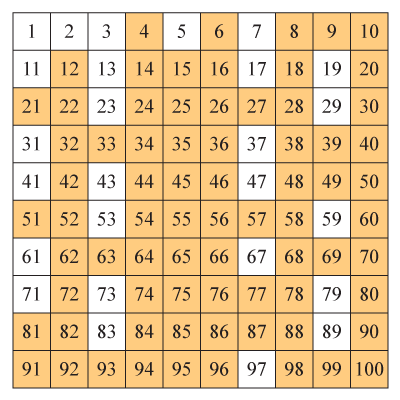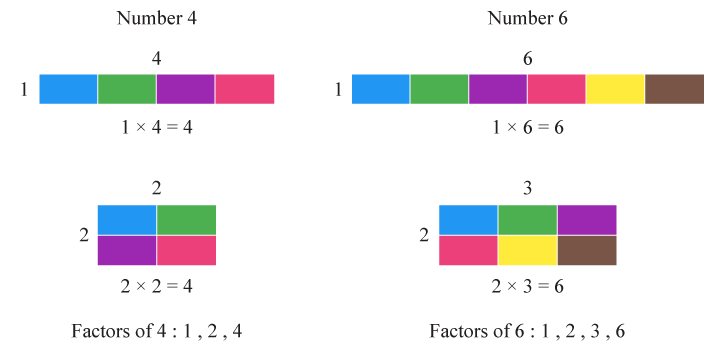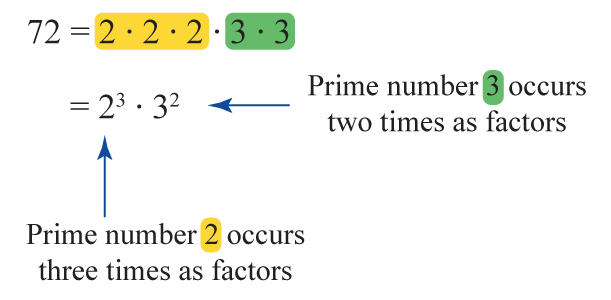# What are Composite Numbers?

What are Composite Numbers?
Go back to  'Numbers'

In this mini lesson, we will explore the world of composite numbers and their characteristics. Let's unlock the composite numbers from 1 to 100. Also learn about the even and odd composite numbers and the smallest and largest composite numbers.

We are sure you will enjoy this mini lesson. Let’s get going!

Here is a list of composite numbers from 1-100. The numbers inside the colour boxes are composite.

##Do you know, why are they called composite?

What about the numbers which are not inside the colour boxes? What are they called?

To get the answer to all these questions and much more, let's begin with meaning of composite numbers.

## Lesson Plan

 1 What are Composite Numbers? 2 Solved Examples on Composite Numbers 3 Interactive Questions on Composite Numbers 4 FREE Downloadable Worksheets on Composite Numbers

## Free PDFs for Offline Revision

Get your copy of Composite Numbers E-book along with Worksheets and Tips and Tricks PDFs for Free!

## What are Composite Numbers?

Composite numbers can be defined as natural numbers that have more than two factors.

Composite numbers are those natural numbers that are not prime and have more than two positive factors.

Natural numbers that are not prime are composite numbers because they are divisible by more than two numbers.

### Examples of Composite Numbers

4, 6, 8, 9, and 10 are the first few composite numbers.

Let's take 4 and 6In the above example,  4 and 6 are called "Composite" numbers because they are  "made by combining other numbers."

This idea is so important and we used it in a theorem called The Fundamental Theorem of Arithmetic.

Let's proceed to understand important features of composite numbers.

### Characteristics of Composite Numbers

A composite number is a positive integer that can be formed by multiplying two smaller positive integers.

Every positive integer is composite, prime, or unit 1, so the composite numbers are evenly divisible by smaller numbers that can be prime, composite, and 1.

For a composite number, there is a minimum of two primes that can be multiplied together to make the number.

### Example:As we see in the image above, while multiplying these positive integers we get a composite number.

## Learn These Along with Composite Numbers!

We are sure you must have gained lot of undertanding about composite numbers. Would you like to explore a bit more?

Here are a few more lessons related to composite numbers. These topics will not only help you master the concept for composite numbers, but also other topics related to it.

 Click on any of the mini lessons you want to explore! PEMDAS Ordinal Numbers Consecutive Numbers Perfect Numbers Co-Prime Numbers Prime Numbers The Fundamental Theorem of Arithmetic

Let's continue our journey and explore different types of composite numbers.

### How to determine the Composite Numbers?

• Find all the factors of a given number.
• If the number has more than two factors, then it is composite.
• The best way to figure out if it is a composite number is to perform the divisibility test.
• The rule states that a number is divisible by any composite number- if it is divisible by each of its factors.
• Performing the divisibility test will help you determine whether or not a number is a prime or composite number.
• If the larger number is divisible by all factors, it is divisible by the composite number.

Want to have some FUN with numbers. We have a simulation for you on composite numbers.

Click on the box and type a number. This simulation helps you to identify a composite number and also the factors of given number.

Let's PLAY !

### Different types of Composite Numbers

The two main types of composite numbers in maths are:

• Odd Composite Numbers
• Even Composite Numbers

### Odd Composite Numbers

All the odd integers which are not prime are odd composite numbers.

Examples of composite odd numbers are 9, 15, 21, 25, 27, etc.

Consider the numbers 1, 2, 3, 4, 9, 10, 11, 12 and 15.

Here 9 and 15 are the odd composites because those two numbers have the odd divisors and satisfy the composite condition.

### Even Composite Numbers

All the even numbers which are not prime are even composite numbers.

Examples of composite even numbers are 4, 6, 8, 10, 12, 14, 16, etc.

Consider the numbers 1, 2, 3, 4, 9, 10, 11, 12 and 15 again.

Here 4, 10, and 12 are the even composites because those two numbers have even divisors and satisfy the composite condition.

Do you know about the smallest composite number? Let's talk about the smallest composite number.

### Smallest Composite Number

A composite number is defined as a number that has divisors other than1 and the number itself.

Start counting : 1, 2, 3, 4, 5, 6, .... so on.

1 is not a composite number because its sole divisor is 1.

2 is not a composite number because it has only two divisors; i.e 1 and the number itself.

3 is not a composite number because it has only two divisors; i.e 1 and the number 3 itself.

Let’s look at number 4...

Its divisors are 1, 2, and 4.

Number 4 satisfies the criteria of a composite number.

So, 4 is the smallest composite number.

Put on your thinking caps and get ready for some interestnig questions on composite numbers!Think Tank
1. Is 21 a composite number?
2. Is 72 a composite number?
3. How many factors does 42 have?
4. How many composite numbers are there between 40 to 50?### What are Composite Numbers from 1 to 100?

There are 74 composite numbers between 1 and 100 (including 1 and 100)

Composite numbers between 1 to 10 4, 6, 8, 9, 10 12, 14, 15, 16, 18, 20 21, 22, 24, 25, 26, 27, 28, 30 32, 33, 34, 35, 36, 38, 39, 40 42, 44, 45, 46, 48, 49, 50 51, 52, 54, 55, 56, 57, 58, 60, 62, 63, 64, 65, 66, 68, 69, 70, 72, 74, 75, 76, 77, 78, 80, 81, 82, 84, 85, 86, 87, 88, 90, 91, 92, 93, 94, 95, 96, 98, 99, 100Tips and Tricks
1. Every composite number has at least 3 factors.
2. Two composite numbers may be coprime or relatively prime.
3. Every even composite number will have 2 as its factor.
4. A perfect square number is always composite.

With this, we are sure that you will be solve any problem based on composite numbers. See, if you can get these right.

## Solved Examples

 Example 1

Is 21 a composite number?

Solution:

Given number is 21

Its factors or divisors are -

1, 3, 7, 21

It has more than two factors.

 $$\textit { ∴ 21 is a composite number}$$
 Example 2

Determine whether 486 is a composite number or not?

Solution:

Given number is 486

Its factors or divisors are -

1, 2, 3, 6, 9, 18, 27, 54, 81, 162, 243, 486

It has more than two factors.

 $$\therefore$$ 486 is a composite number

## Interactive Questions on Consecutive Numbers

Here are a few activities for you to practice. Select/Type your answer and click the "Check Answer" button to see the result.

## Let’s Summarize

The mini-lesson was aimed at helping you learn about composite numbers and their characteristics. Hope you enjoyed learning about them and exploring various types of composite numbers.

We would love to hear from you. Drop us your comments in the chat and we would be happy to help.

At Cuemath, our team of math experts is dedicated to making learning fun for our favorite readers, the students! Through an interactive and engaging learning-teaching-learning approach, the teachers explore all angles of a topic. Be it worksheets, online classes, doubt sessions, or any other form of relation, it’s the logical thinking and smart learning approach that we at Cuemath believe in.

## 1. How to find composite numbers?

To find whether a given number is a composite, we just find its number of factors.

If it has more than 2 factors then the number is composite. You can find the examples under "Solved Examples”.

## 2. Is 2 a composite number?

A composite number has more than two factors.

In this case, 2 has only two factors i.e. 1 and 2.

Therefore, two is a prime number, not a composite number.

## 3. What are the composite numbers between 1 and 100?

The composite numbers between 1 and 100 are-

4, 6, 8, 9, 10, 12, 14, 15, 16, 18, 20, 21, 22, 24, 25, 26, 27, 28, 30, 32, 33, 34, 35, 36, 38, 39, 40, 42, 44, 45, 46, 48, 49, 50, 51, 52, 54, 55, 56, 57, 58, 60, 62, 63, 64, 65, 66, 68, 69, 70, 72, 74, 75, 76, 77, 78, 80, 81, 82, 84, 85, 86, 87, 88, 90, 91, 92, 93, 94, 95, 96, 98, 99, 100.

## 4. What is a composite number and prime number?

When a number can be divided up exactly it is known as Composite Number.

When a number cannot be divided up exactly it is known as the Prime Number.

Composite Numbers- Ebook
Ebook
Composite Numbers -Tips and Tricks
Composite Numbers